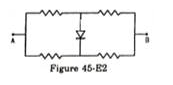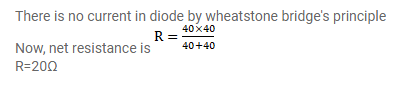# Each of the resistance shown in figure (45-E2) has a value of

Question:

Each of the resistance shown in figure (45-E2) has a value of $20 \Omega$. Find the equivalent resistance between A and B. Does it depends on whether the point $\mathrm{A}$ or $\mathrm{B}$ is at higher potential?Solution: